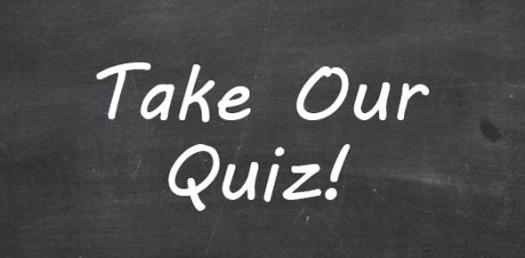# Network Devices - Week 5

33 Questions | Attempts: 185
ShareSettings• 1.
• 2.
• 3.
• 4.
• 5.
What layer of the internet model do hubs operate in?
• A.

Application

• B.

Network

• C.

Physical

• D.

• 6.
What layer of the internet model do routers operate in?
• A.

Application

• B.

• C.

Network

• D.

Physical

• 7.
What layer of the internet model do switches operate in?
• A.

Application

• B.

• C.

Network

• D.

Physical

• 8.
When hosts are connected, a(n)  __________ is created.
• A.

Network

• B.

Internet

• C.

Access Point

• D.

Bridge

• 9.
When networks are connected, a(n)  __________ is created.
• A.

Network

• B.

Internet

• C.

Access Point

• D.

Bridge

• 10.
What is the diagram an example of:
• A.

Repeater

• B.

Hub

• C.

Router

• D.

Bridge

• E.

Switch

• 11.
What is the diagram an example of:
• A.

Repeater

• B.

Hub

• C.

Router

• D.

Bridge

• E.

Switch

• 12.
What is the diagram an example of:
• A.

Repeater

• B.

Hub

• C.

Router

• D.

Bridge

• E.

Switch

• 13.
What is the diagram an example of:
• A.

Repeater

• B.

Hub

• C.

Router

• D.

Bridge

• E.

Switch

• 14.
What is the diagram an example of:
• A.

Repeater

• B.

Hub

• C.

Router

• D.

Bridge

• E.

Switch

• 15.
Which layers does a Link Layer Switch operate in:
• A.

Application

• B.

Transport

• C.

Network

• D.

• E.

Physical

• 16.
A "muti-port repeater" is another name for a(n)
• A.

Repeater

• B.

Hub

• C.

Router

• D.

Bridge

• E.

Switch

• 17.
This device has become the "backbone" of the internet:
• A.

Repeater

• B.

Hub

• C.

Router

• D.

Bridge

• E.

Switch

• 18.
Select all that apply for the term: Amplitude
• A.

Analog wave's strength

• B.

Number of times amplitude cycles over fixed time period

• C.

Is measured in hertz (Hz)

• D.

Distance between corresponding wave cycle points

• E.

Inversely proportional to frequency

• F.

Expressed in meters or feet

• G.

Wave's progress over time in relationship to fixed point

• 19.
Select all that apply for the term: Phase
• A.

Analog wave's strength

• B.

Number of times amplitude cycles over fixed time period

• C.

Is measured in hertz (Hz)

• D.

Distance between corresponding wave cycle points

• E.

Inversely proportional to frequency

• F.

Expressed in meters or feet

• G.

Wave's progress over time in relationship to fixed point

• 20.
Select all that apply for the term: Wavelength
• A.

Analog wave's strength

• B.

Number of times amplitude cycles over fixed time period

• C.

Is measured in hertz (Hz)

• D.

Distance between corresponding wave cycle points

• E.

Inversely proportional to frequency

• F.

Expressed in meters or feet

• G.

Wave's progress over time in relationship to fixed point

• 21.
Select all that apply for the term: Frequency
• A.

Analog wave's strength

• B.

Number of times amplitude cycles over fixed time period

• C.

Measured in hertz (Hz)

• D.

Distance between corresponding wave cycle points

• E.

Inversely proportional to frequency

• F.

Expressed in meters or feet

• G.

Wave's progress over time in relationship to fixed point

• 22.
A signal that is continuous in time and value is called a(n) ___________ signal
• 23.
A signal that is discrete in time and value is called a(n) ___________ signal
• 24.
Measures amount of data transmitted during given time period
• A.

Throughput

• B.

Bandwidth

• C.

Data Modulation

• D.

Channel

• 25.
Measures difference between highest and lowest frequencies medium can transmit
• A.

Throughput

• B.

Bandwidth

• C.

Data Modulation

• D.

ChannelBack to top